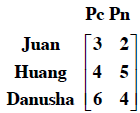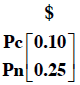### Home > A2C > Chapter 7 > Lesson 7.3.4 > Problem7-203

7-203.

Juan, Huang, and Danusha have pencils and pens in their backpacks. Juan has $3$ pencils and $2$ pens, Huang has $4$ pencils and $5$ pens, and Danusha has $6$ pencils and $4$ pens.

1. Represent this information in a $3\text{-by-}2$ matrix. Label rows and columns.2. Each pencil is worth $10$ cents, and each pen is worth $25$cents. Represent this information in a matrix in such a way that you can multiply the first matrix by this one. Label rows and columns.3. Find the product matrix and interpret the $2$, $1$ entry.

The 2,1 entry is 1.65, and represents the total value of Huang's pens and pencils.